# Forces - calculating the net force

## Homework Statement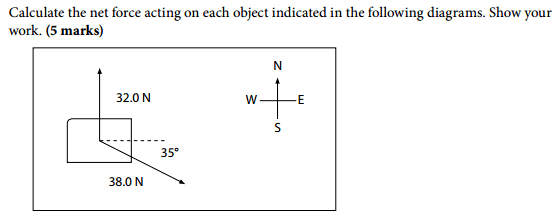## Homework Equations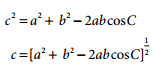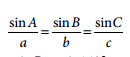## The Attempt at a Solution

c = ([32.0 N ]^2 + [38.0 N]^2 - 2 (32.0 N)(38.0 N) cos70° ) 1/2

c = 40.5 N (is this correct?)

SinA / 38.0 N = Sin110° /40.5 N (im unsure how to solve this part)
A = ??
net force = 40.5 N (East ??? North)

I am very confused and I have no idea where to go from here
please explain what im supposed to do, and if my answer is correct
thanks

## Answers and Replies

It will make more sense if you represent the forces as a vector.

You have the 32N component in the +z direction and then you have the 38N that lies in the x-y plane. Using basic trig and treating it as if if it were a normal triangle, you can resolve the x and y components.

After that, take the magnitude of the vector to get the total force.

haruspex
Science Advisor
Homework Helper
Gold Member
2020 Award
@alexandria , where do you get 70 degrees from?

You have the 32N component in the +z direction and then you have the 38N that lies in the x-y plane. Using basic trig and treating it as if if it were a normal triangle, you can resolve the x and y components.
I interpret the diagram as purely 2-dimensional. Note the compass.

•CAT 2
The lesson that im doing provides a similar question and answer, i tried to follow it for the question that I am answering, but i dont know where i went wrong. Here is the question from the lesson. Could you explain how i should answer my question using the qts below as a guideline. I undestand what you mean by the x and y plane, however the lesson wants me to follow this method: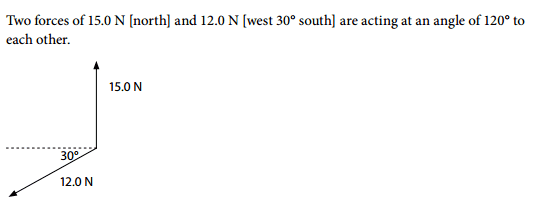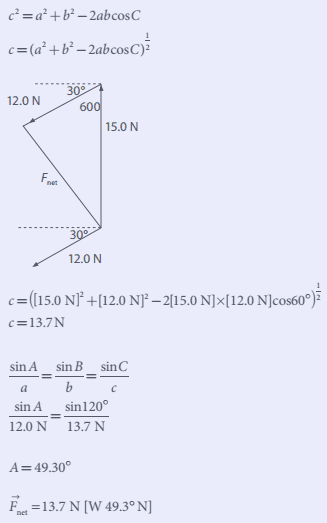i need to know how to use this same method to answer my question. please help
thanks

@haruspex
i got 70 degrees by adding 35 + 35
i think i did that wrong, and its supposed to be cos55
what do you think?

haruspex
Science Advisor
Homework Helper
Gold Member
2020 Award
@haruspex
i got 70 degrees by adding 35 + 35
i think i did that wrong, and its supposed to be cos55
what do you think?
There is only one angle of 35 degrees in the diagram, so why are you adding another 35?
I suspect that you misunderstand how 60 degrees was arrived at in the worked example. It was not by adding 30 and 30.
Look at your diagram. What is the angle between the two vectors?

•CAT 2
can you elaborate, im very confused?
but anyways, here is my second attempt to my question:
c = ([32.0 N ]^2 + [38.0 N]^2 - 2 (32.0 N)(38.0 N) cos55° ) 1/2 (i got 55 degrees, by dividing 110 in half)

c = 32.7 N (is this correct?)

SinA / 38.0 N = Sin55° /32.7 N
A = 72 degrees
net force = 32.7 N (North 72 degrees East)

haruspex
Science Advisor
Homework Helper
Gold Member
2020 Award
c = ([32.0 N ]^2 + [38.0 N]^2 - 2 (32.0 N)(38.0 N) cos55° ) 1/2 (i got 55 degrees, by dividing 110 in half)
But where did you get 110 from?!
Look at the diagram. The 38N force points down and to the right. Above it there is a dashed line. What is the angle between the 38N force and the dashed line?
What is the angle between the dashed line and the 32N vector?

•CAT 2
the angle between the 38N force and the dashed line is 35 degrees
the angle between the dashed line and the 32N vector is 90 degrees

haruspex
Science Advisor
Homework Helper
Gold Member
2020 Award
the angle between the 38N force and the dashed line is 35 degrees
the angle between the dashed line and the 32N vector is 90 degrees
Right. So what is the angle between the two forces?

•CAT 2
so 90 + 35
the angle between the two force would be 125 degrees
is this the angle i would use in my solution

haruspex
Science Advisor
Homework Helper
Gold Member
2020 Award
so 90 + 35
the angle between the two force would be 125 degrees
is this the angle i would use in my solution
Yes, 125 degrees, but we're not quite ready to apply the formula.
I don't think you understand the parallelogram of forces method used here, so I'll spell it out for you.
You have two forces acting at a point, call it O. The forces are represented by lines OA and OB, showing the magnitudes and directions.
To find the resultant, you need to construct a fourth point, C, such that OACB forms a parallelogram. Can you draw that?
If the angle AOB is 125 degrees, what is the angle OAC?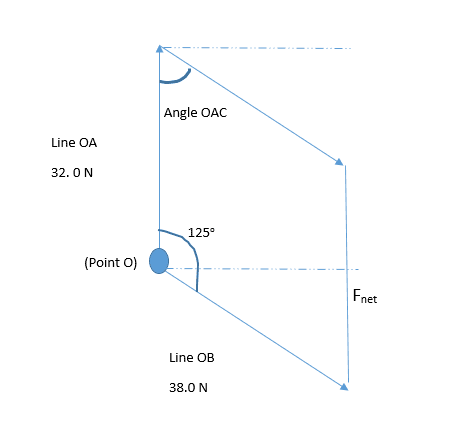is this what you mean. Line OA and OB represent the two forces acting at point O. is this what you mean by a parallelogram. I dont know how to solve for angle OAC

haruspex
Science Advisor
Homework Helper
Gold Member
2020 Award
View attachment 97351
is this what you mean. Line OA and OB represent the two forces acting at point O. is this what you mean by a parallelogram. I dont know how to solve for angle OAC
Yes, that's the right diagram.
You have a straight line OA meeting two parallel lines OB, AC. The relationship between angles AOB, OAC is very basic geometry.

•CAT 2
im still not getting it, how would i solve for OAC?
does it have anything to do with using these equations: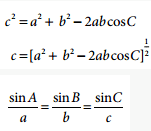and once i solve for OAC would that give me the angle im supposed to use to solve for the resultant

wait would angle OAC be 55 degrees
90-35 = 55

haruspex
Science Advisor
Homework Helper
Gold Member
2020 Award
im still not getting it, how would i solve for OAC?
Let's try something you might remember.
The angle OAC is obviously the same as OBC. The line OB is the hypotenuse of a right angled triangle involving the dashed line. Your original 35 degrees is an angle of that triangle. So what is angle OBC?

•CAT 2
haruspex
Science Advisor
Homework Helper
Gold Member
2020 Award
wait would angle OAC be 55 degrees
90-35 = 55
Yes.

•CAT 2
ok, so if angle OAC is 55 degrees, what am i using in my answer to solve for the net force: 55 degrees or 125 degrees
im confused, what do these numbers represent?

haruspex
Science Advisor
Homework Helper
Gold Member
2020 Award
ok, so if angle OAC is 55 degrees, what am i using in my answer to solve for the net force: 55 degrees or 125 degrees
im confused, what do these numbers represent?
OC represents the resultant. When using the cosine formula to find the length of a side from the other two, which angle do you use?
If you are trying to find the length of side OC in this problem, do you want the 125 or the 55?

•CAT 2
to find the length of side OC, i would use 125 degrees, so using the cosine formula:
c = ([32.0 N ]^2 + [38.0 N]^2 - 2 (32.0 N)(38.0 N) cos125° ) 1/2

haruspex
Science Advisor
Homework Helper
Gold Member
2020 Award
to find the length of side OC, i would use 125 degrees, so using the cosine formula:
c = ([32.0 N ]^2 + [38.0 N]^2 - 2 (32.0 N)(38.0 N) cos125° ) 1/2
Go for it!

c = ([32.0 N ]^2 + [38.0 N]^2 - 2 (32.0 N)(38.0 N) cos125° ) 1/2
c = 62.1 N (Is this correct?)
is this how i would solve for the angle of the net force
SinA / 38.0 N = Sin125° /62.1 N

haruspex
Science Advisor
Homework Helper
Gold Member
2020 Award
to find the length of side OC, i would use 125 degrees, so using the cosine formula:
c = ([32.0 N ]^2 + [38.0 N]^2 - 2 (32.0 N)(38.0 N) cos125° ) 1/2
Rats! I didn't read that post properly before. Why have you gone back to 125 degrees? I thought we agreed that 55 degrees was the angle to be used.

lol ok, so 55 degrees would be used to find the length of OC
c = ([32.0 N ]^2 + [38.0 N]^2 - 2 (32.0 N)(38.0 N) cos55° ) 1/2
c= 32.9 N (right?)
then is this how i would solve for the angle of the net force:
SinA / 38.0 N = Sin55° /32.9 N